# Convergence

(redirected from positive convergence)
Also found in: Dictionary, Thesaurus, Medical, Financial.

## convergence

[kən′vər·jəns]
(anthropology)
Independent development of similarities between unrelated cultures.
(evolution)
Development of similarities between animals or plants of different groups resulting from adaptation to similar habitats.
(electronics)
A condition in which the electron beams of a multibeam cathode-ray tube intersect at a specified point, such as at an opening in the shadow mask of a three-gun color television picture tube; both static convergence and dynamic convergence are required.
(geology)
Diminution of the interval between geologic horizons.
(hydrology)
The line of demarcation between turbid river water and clear lake water.
(mathematics)
The property of having a limit for infinite series, sequences, products, and so on.
(meteorology)
The increase in wind setup observed beyond that which would take place in an equivalent rectangular basin of uniform depth, caused by changes in platform or depth.
(neuroscience)
The coming together of a group of afferent nerves upon a motoneuron of the ventral horn of the spinal cord.
(oceanography)
A condition in the ocean in which currents or water masses having different densities, temperatures, or salinities meet; results in the sinking of the colder or more saline water.
(physics)
The intersection of light beams or particles within a small region, or the narrowing of a single beam so that it passes through a small region.
McGraw-Hill Dictionary of Scientific & Technical Terms, 6E, Copyright © 2003 by The McGraw-Hill Companies, Inc.
The following article is from The Great Soviet Encyclopedia (1979). It might be outdated or ideologically biased.

## Convergence

in biology, the evolutionary development of similar characteristics by organisms of distantly related groups; the acquisition of a similar structure as a result of similar environments and parallel natural selection. Owing to convergence, organs that perform the same function in different organisms acquire a similar structure. An example is the convergent resemblance of the shape of the body and fins of the extinct marine reptile ichthyosaurus, the mammal dolphin, and fish. The resemblances resulting from convergent evolution are superficial.

A. A. MAKHOTIN

## Convergence

in linguistics, the assimilation of elements of a language (for example, sounds) or various languages (the opposite of divergence).

Convergence of sounds often leads to the coincidence of two former phonemes in one; in some instances this is accounted for by a physical change in the sound (for example, the coincidence of the Proto-Germanic phonemes [θ] and [t] in the phoneme [t] in the Scandinavian languages: Swedish torn, English thorn); in others it is caused only by the internal reconstruction of the phonological system (for example, the coincidence of Russian [t] and [y] in one phoneme as a result of phonemization of the opposition of hard and soft consonants).

Convergence of languages includes instances of the development by two or several languages of similar characteristics not to be explained by the common origin of these languages, as a result of territorial proximity, cultural ties, and the like.

## Convergence

in physiology, the coordinated movement of the visual axes of the two eyes toward a fixed object. It occurs as a result of the contraction of the medial rectus oculi and the partial contraction of the superior and inferior recti oculi of both eyes. Convergence is accompanied by contraction of the pupils (miosis) and the tension of accommodation. The unit of convergence is the meter angle, or the angle that the visual line forms with a perpendicular erected from the middle of the bridge of the nose when the eyes are fixed on a point at a distance of 1 m. For example, at two meter angles the eyes converge at a point at a distance of 0.5 m. Intensified convergence when there is considerable farsightedness (presbyopia) and weakened convergence when there is nearsightedness (myopia) may lead to strabismus.

## Convergence

in mathematics, the property, for a variable quantity, of having a limit. We may speak of the convergence of an infinite sequence, an infinite series, an infinite product, a continued fraction, an integral, and so on. The concept of convergence arises in the study of a mathematical entity, for example, when there can be constructed a sequence of, in some sense, simpler entities that approach the given entity—that is, that have the given entity as their limit. Thus, the sequence of perimeters of regular polygons inscribed in a circle may be used to compute the circumference of the circle, and the sequences of partial sums of the series representing certain functions may be used to compute the values of the functions.

The convergence of a sequence {an}, n = 1,2,..., means that a finite limit exists for the sequence;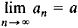In the case of a seriesconvergence means that the sequence of partial sums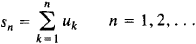of the series has a finite limit. The convergence of an infinite product b1b2 ... bn ... means that the sequence of finite products pn = b1b2 ... bn, n = 1, 2, ..., has a nonzero finite limit. For an integral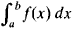of a function f(x) that is integrable over any finite interval [a, b], convergence means that when b → + ∞, the integrals have a finite limit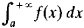which is known as an improper integral.

The property of the convergence of various mathematical entities plays an important role in both theoretical and applied mathematics. Quantities or functions are often represented by means of convergent series. For example, the base e of the natural logarithms can be expanded in a convergent series: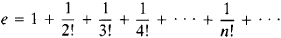The function sin x can be expanded in a series that converges for all x;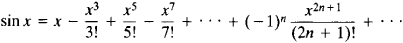Such series may be used to approximate the corresponding quantities or functions. For this purpose, it is sufficient to take the sum of the initial terms of the series; the greater the number of terms taken, the greater the accuracy of the value obtained.

Different series expansions can be found for the same quantities or functions. For example,To reduce the number of calculations and, consequently, to save time and reduce the number of errors, it is advisable in practical calculations to select from among the available series the series that converges most rapidly. Suppose two convergent series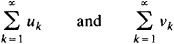are given and rn = un + 1 + un + 2 + ... and ρn = vn + 1 + vn + 2 + ... are their remainders after the nth term. The first series is said to converge more rapidly than the second if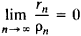For example, the series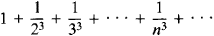converges more rapidly than the seriesOther concepts of more rapidly converging series are also used. Various methods exist for improving the convergence of series. By means of such methods, a given series can be transformed into a more rapidly converging one. The concept of more rapid convergence is introduced for improper integrals in much the same way as for series; methods of improving the convergence of improper integrals also exist.

The concept of convergence plays an important role in the solution of algebraic, differential, and integral equations, particularly in the finding of approximate numerical solutions. For example, the method of successive approximations can be used to obtain a sequence of functions that converges to the corresponding solution of a given ordinary differential equation. The existence of a solution under certain conditions is thereby proved; at the same time, a method is obtained for computing the solution to the desired accuracy. A well-developed theory of different convergent finite-difference methods exists for the numerical solution of both ordinary and partial equations (seeNET-POINT METHOD). Extensive use is made of computers in the practical approximate solution of equations.

If the terms an of a sequence {an} are represented on a number line, the convergence of the sequence to a means that the distance between the points an and a becomes, and remains, arbitrarily small with increasing n. By using such a formulation, the concept of convergence can be extended to sequences of points in the plane, in space, and in more general entities. It is required here that a concept of distance can be defined that has all the usual properties of the distance between points in space. The notion of convergence can thus be extended to sequences of such entities as vectors, matrices, functions, and geometric figures (seeMETRIC SPACE). If a sequence {an} converges to a, then outside any neighborhood of a there lie only a finite number of terms of the sequence. This formulation permits the concept of convergence to be extended to sets of more general types of quantities where the concept of neighborhood is defined.

Mathematical analysis makes use of various types of convergence of a sequence of functions {fn(x)} to a function f(x) (on some set M). If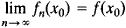for every point x0 in M, we speak of convergence everywhere; if this equality is violated only by points forming a set of measure zero (see), we speak of convergence almost everywhere. In spite of its naturalness, the concept of convergence everywhere has many undesirable features. For example, a sequence of continuous functions may converge everywhere to a discontinuous function; the convergence of the functions fn(x) to f(x) everywhere does not in general imply that the integrals of fn(x) converge to the integral of f(x). The concept of uniform convergence, which is free of these shortcomings, was therefore introduced. A sequence {fn(x) is said to converge uniformly to f(x) on the set M if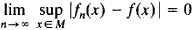This type of convergence corresponds to the following definition of the distance between the functions f(x) and ϕ(x):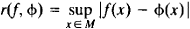D. F. Egorov proved that if a sequence of measurable functions converges almost everywhere on a set M, a subsequence of arbitrarily small measure may be removed from M so that uniform convergence holds for the remaining portion.

The concept of convergence in the mean is used extensively in the theory of, for example, integral equations and orthogonal series. A sequence {fn(x)} converges in the mean of order two to f(x) on the interval [a, b] ifMore generally, a sequence {fn(x)} converges in the mean of order p to f(x) if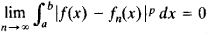This type of convergence corresponds to the following definition of the distance between two functions:Uniform convergence on a finite closed interval implies convergence in the mean of any order p. The sequence of partial sums of the expansion of a square integrable function ϕ(x) in a series of normalized orthogonal functions may diverge everywhere, but such a series always converges in the mean of order two to φ(x).

Other types of convergence are also considered, for example, convergence in measure. Here, for any ∈ > 0, the measure of the set of points at which ǀfn(x) – f(x)ǀ < ∈ approaches zero as n increases. Weak convergence is defined as follows:for any square integrable function ϕ(x). For example, the sequence of functions sin x, sin 2x, ..., sin nx, ..., converges weakly to zero on the interval [–π, π], since for any square integrable function ϕ(x) the coefficients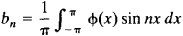of the Fourier series approach zero.

The above concepts, along with many other concepts, of the convergence of a sequence of functions are systematically studied in functional analysis, which deals with various linear spaces with a specified norm (distance from zero)—that is, Banach spaces. Concepts of the convergence of functionals, operators, and so on may be introduced for these spaces when the norm is defined in an appropriate manner. Weak convergence, defined by the condition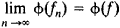for all linear functionals, is considered in Banach spaces, as is strong convergence. The above definition of weak convergence of functions corresponds to the norm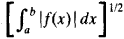In modern mathematics convergence in partially ordered sets is also considered. The concepts of convergence with probability 1 and convergence in probability are used in probability theory for sequences of random variables.

Such mathematicians of antiquity as Euclid and Archimedes in effect made use of infinite series to find areas and volumes. These mathematicians employed rigorous arguments along the lines of the method of exhaustion to prove the convergence of series. The term “convergence” was first used with respect to series in 1668 by J. Gregory in his study of certain methods of computing the area of circles and hyperbolic sectors. The mathematicians of the 17th century generally had a clear notion of the convergence of the series they employed. From the modern standpoint, however, their convergence proofs lacked rigor. In 18th-century analysis, extensive use was made, particularly by L. Euler, of series that were known to diverge. Numerous misunderstandings and errors subsequently resulted, which were not eliminated until the development of a clear theory of convergence. On the other hand, the work of the 18th-century mathematicians with divergent series anticipated the modern theory of the summation of divergent series.

Rigorous methods of investigating the convergence of series were developed in the 19th century by such mathematicians as A. Cauchy, N. Abel, K. Weierstrass, and B. Bolzano. The concept of uniform convergence was introduced by G. Stokes. Further extensions of the concept of convergence were associated with the development of the theory of functions, functional analysis, and topology.

### REFERENCES

Il’in, V. A., and E. G. Pozniak. Osnovy matematicheskogo analiza, 3rd ed., vols. 1–2. Moscow, 1971–73.
Kudriavtsev, L. D. Matematicheskii analiz, 2nd ed., vols. 1–2. Moscow, 1970.
Nikol’skii, S. M. Kurs matematicheskogo analiza, vols. 1–2. Moscow, 1973.

## convergence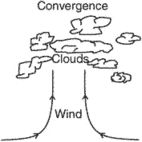i. The condition that exists when the distribution of winds within a given area is such that there is a net horizontal inflow of air into the area. In convergence at lower levels, the removal of the resulting excessive air is accompanied by upward movement. Clouds and rain are likely to occur in areas of low-level convergent winds.
ii. As it relates to aerial photography interpretation, it means the turning of two eyes while viewing an object. The convergence of the eyes is a clue to distance. The eyes converge more for nearby points and less for farther points.